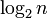# Unitriangular matrix group:UT(3,Z4)

View a complete list of particular groups (this is a very huge list!)[SHOW MORE]

## Definition

### As a matrix group

This group is defined as the unitriangular matrix group of degree three over ring:Z4. Explicitly, it is the group (under matrix multiplication) of upper-triangular$3 \times 3$ unipotent matrices over the ring$\mathbb{Z}/4\mathbb{Z}$, i.e., matrices of the form:$\left\{ \begin{pmatrix} 1 & a_{12} & a_{13} \\ 0 & 1 & a_{23} \\ 0 & 0 & 1 \\\end{pmatrix} : a_{12}, a_{13}, a_{23} \in \mathbb{Z}/4\mathbb{Z} \right\}$

### Definition by presentation

This group is given by the following presentation:$G := \langle x,y,z \mid x^4 = y^4 = z^4 = e, [x,y] = z, xz = zx, yz = zy\rangle$

Here's how this presentation relates to the matrix description:

We can relate this with the matrix group definition by setting:$x = \begin{pmatrix} 1 & 1 & 0 \\ 0 & 1 & 0 \\ 0 & 0 & 1 \\\end{pmatrix}, \qquad y = \begin{pmatrix} 1 & 0 & 0 \\ 0 & 1 & 1 \\ 0 & 0 & 1 \\\end{pmatrix}, \qquad z = \begin{pmatrix} 1 & 0 & 1 \\ 0 & 1 & 0 \\ 0 & 0 & 1 \\\end{pmatrix}$

## Arithmetic functions

Want to compare and contrast arithmetic function values with other groups of the same order? Check out groups of order 64#Arithmetic functions

### Basic arithmetic functions

Function Value Similar groups Explanation
underlying prime of p-group 2
order (number of elements, equivalently, cardinality or size of underlying set) 64 groups with same order As$UT(n,R), n = 3, |R| = 4$:$|R|^{n(n-1)/2} = 4^{3(2)/2} = 4^3 = 64$
prime-base logarithm of order 6 groups with same prime-base logarithm of order
max-length of a group 6 max-length of a group equals prime-base logarithm of order for group of prime power order
chief length 6 chief length equals prime-base logarithm of order for group of prime power order
composition length 6 composition length equals prime-base logarithm of order for group of prime power order
exponent of a group 8 groups with same order and exponent of a group | groups with same exponent of a group
prime-base logarithm of exponent 3 groups with same order and prime-base logarithm of exponent | groups with same prime-base logarithm of order and prime-base logarithm of exponent | groups with same prime-base logarithm of exponent
nilpotency class 2 groups with same order and nilpotency class | groups with same prime-base logarithm of order and nilpotency class | groups with same nilpotency class As$UT(n,R)$ for$R$ commutative:$n - 1 = 3 - 1 = 2$
derived length 2 groups with same order and derived length | groups with same prime-base logarithm of order and derived length | groups with same derived length As$UT(n,R)$ for$R$ commutative: smallest integer greater than or equal to$\log_2n$
Frattini length 3 groups with same order and Frattini length | groups with same prime-base logarithm of order and Frattini length | groups with same Frattini length
minimum size of generating set 2 groups with same order and minimum size of generating set | groups with same prime-base logarithm of order and minimum size of generating set | groups with same minimum size of generating set
rank of a p-group 3 groups with same order and rank of a p-group | groups with same prime-base logarithm of order and rank of a p-group | groups with same rank of a p-group
normal rank of a p-group 3 groups with same order and normal rank of a p-group | groups with same prime-base logarithm of order and normal rank of a p-group | groups with same normal rank of a p-group
characteristic rank of a p-group 3 groups with same order and characteristic rank of a p-group | groups with same prime-base logarithm of order and characteristic rank of a p-group | groups with same characteristic rank of a p-group

## Elements

Further information: element structure of unitriangular matrix group:UT(3,Z4)

## Linear representation theory

Further information: linear representation theory of unitriangular matrix group:UT(3,Z4)

Item Value
Degrees of irreducible representations over a splitting field (such as$\mathbb{C}$ or$\overline{\mathbb{Q}}$) 1 (16 times), 2 (4 times), 4 (2 times)
maximum: 4, lcm: 4, number: 22, sum of squares: 64

## GAP implementation

### Group ID

This finite group has order 64 and has ID 18 among the groups of order 64 in GAP's SmallGroup library. For context, there are groups of order 64. It can thus be defined using GAP's SmallGroup function as:

SmallGroup(64,18)

For instance, we can use the following assignment in GAP to create the group and name it$G$:

gap> G := SmallGroup(64,18);

Conversely, to check whether a given group$G$ is in fact the group we want, we can use GAP's IdGroup function:

IdGroup(G) = [64,18]

or just do:

IdGroup(G)

to have GAP output the group ID, that we can then compare to what we want.

### Description as a matrix group

This description uses the functions SL, ZmodnZ, IsZero, and IsOne.

gap> L := SL(3,ZmodnZ(4));;
gap> G := Group(Filtered(L,x -> ForAll([x,x,x],IsOne) and ForAll([x,x,x],IsZero)));;
gap> IdGroup(G);
[ 64, 18 ]

### Description by presentation

gap> F := FreeGroup(3);
<free group on the generators [ f1, f2, f3 ]>
gap> G := F/[F.1^4,F.2^4,F.3^4,F.1*F.3*F.1^(-1)*F.3^(-1),F.1*F.2*F.1^(-1)*F.2^(-1),F.2*F.3*F.2^(-1)*F.3^(-1)*F.1^(-1)];
<fp group on the generators [ f1, f2, f3 ]>
gap> IdGroup(G);
[ 64, 18 ]